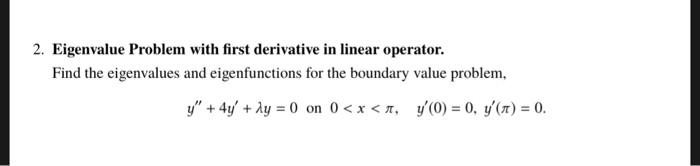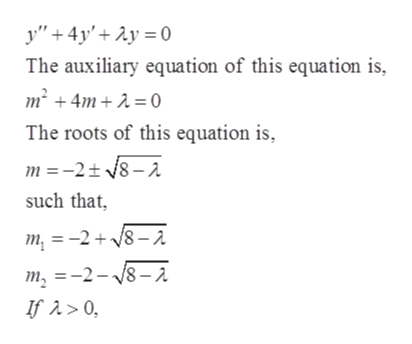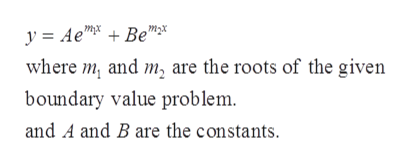# 2. Eigenvalue Problem with first derivative in linear operator.Find the eigenvalues and eigenfunctions for the boundary value problem,y"+4yA 0 on 0

Question
49 viewshelp_outlineImage Transcriptionclose2. Eigenvalue Problem with first derivative in linear operator. Find the eigenvalues and eigenfunctions for the boundary value problem, y"+4yA 0 on 0
check_circle

Step 1

The given boundary value problem,

Step 2

Find the eigen values and eigenfunctions of the given boundary value problem in the following.help_outlineImage Transcriptionclosey"4yAy0 The auxiliary equation of this equation is m24m 0 The roots of this equation is, m -2t8-2 such that m =-28-a m, -2-8-2 fullscreen
Step 3

Therefore, the solution o...help_outlineImage Transcriptionclosey Ae"Be"* where m and m, are the roots of the given boundary value problem. and A and B are the constants. fullscreen

### Want to see the full answer?

See Solution

#### Want to see this answer and more?

Solutions are written by subject experts who are available 24/7. Questions are typically answered within 1 hour.*

See Solution
*Response times may vary by subject and question.
Tagged in

### Math